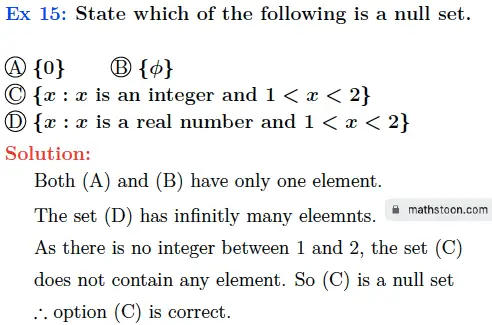# SN Dey Class 11 Set Theory Multiple Choice Questions Solutions

SN Dey Class 11 Solutions | SN Dey Class 11 Set Theory Solutions | Class 11 Set Theory MCQ | SN Dey Solutions | S N Dey Math Solutions | Set Theory S N Dey Solutions | WBCHSE Class 11 Math Solutions | Set theory objective questions

## SN Dey Class 11 Set Theory MCQ Solutions

Choose the correct option:

Ex 1: The number of subsets in a set consisting of four distinct elements is-

(A) 4   (B) 8  (C) 16   (D) 64

We know that the number of subsets in a set consisting of n distinct elements is equal to 2n.

= 2= 16.

∴ option (C) is correct.

Ex 2: The number of proper subsets in a set consisting of five distinct elements is-

(A) 5   (B) 10  (C) 32   (D) 31

We know that the number of proper subsets in a set consisting of n distinct elements is equal to 2n-1.

= 25-1= 32-1 = 31.

∴ option (D) is correct.

Ex 3: If x ∈ A ⇒ x ∈ B then –

(A) A=B   (B) A⊂B  (C) A⊆B   (D) B⊆A

As x ∈ A ⇒ x ∈ B, then we must have that either A is a proper subset of B or they are equal. So the answer is A ⊆ B.

∴ option (C) is correct.

Ex 4: If A⊆B and B⊆A then –

(A) A=Φ   (B) A∩B=Φ  (C) A=B   (D) None of these

As A⊆B and B⊆A, then it follows that A=B.

So option (C) is correct.

Ex 5: For two sets A and B, if A∪B = A∩B then –

(A) A⊆B   (B) B⊆A  (C) A=B   (D) None of these

Let  then

As

…..(i)

Similarly, if  then,  =

Thus, we get that B…..(ii)

So from (i) and (ii), we obtain that

∴ option (C) is correct.

Ex 6: A – B = Φ iff – [Council Sample Question ’13]

(A) A≠B   (B) A⊂B  (C) B⊂A   (D) A∩B=Φ

We know that A-B ={x: x ∈ A but x ∉B}. Thus A-B=Φ means that A is fully contained in B, that is, A⊂B.

∴ option (B) is correct.

Ex 7: If A∩B = B then –

(A) A⊆B   (B) B⊆A  (C) A=B   (D) A=Φ

As B=A∩B, we have B ⊆ A∩B.

⇒ B ⊆ A and B ⊆ B.

⇒ B ⊆ A is true.

∴ option (B) is correct.

Ex 8: If A and B are two disjoint sets then n(A∪B)=

(A) n(A)+n(B)   (B) n(A)-n(B)  (C) 0   (D) None of these

As A and B are disjoint sets, so we have A∩B = Φ. Thus n(A∩B)=0.

Now, we know that

n(A∪B) = n(A)+n(B)-n(A∩B)

= n(A)+n(B)+0

= n(A)+n(B)

∴ option (A) is correct.

Ex 9: For any two sets A and B, n(A)+n(B)-n(A∩B)=

(A) n(A∪B)   (B) n(A)-n(B)  (C) Φ   (D) None of these

As n(A∪B) = n(A)+n(B)+n(A∩B), the option (A) is correct.

Ex 10: The dual of A∪U = U is-

(A) A∪U=U   (B) A∪Φ=Φ  (C) A∪Φ=A   (D) A∩Φ=Φ

The dual of A∪U = U is A∩Φ=Φ.

∴ option (D) is correct.

Ex 14: State which of the is the set of factors of the number 12-

(A) {2, 3, 4, 6}   (B) {2, 3, 4, 6, 12}

(C) {2, 3, 4, 8, 6} (D) {1, 2, 3, 4, 6, 12}

The factors of 12 are 1, 2, 3, 4, 6, and 12.

∴ option (D) is correct.SN Dey All Chapter Solutions

Share via: